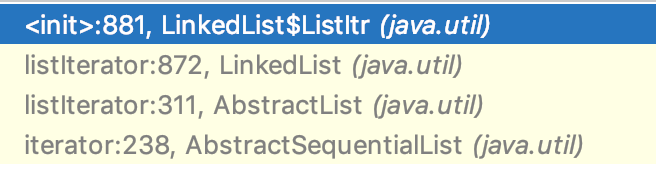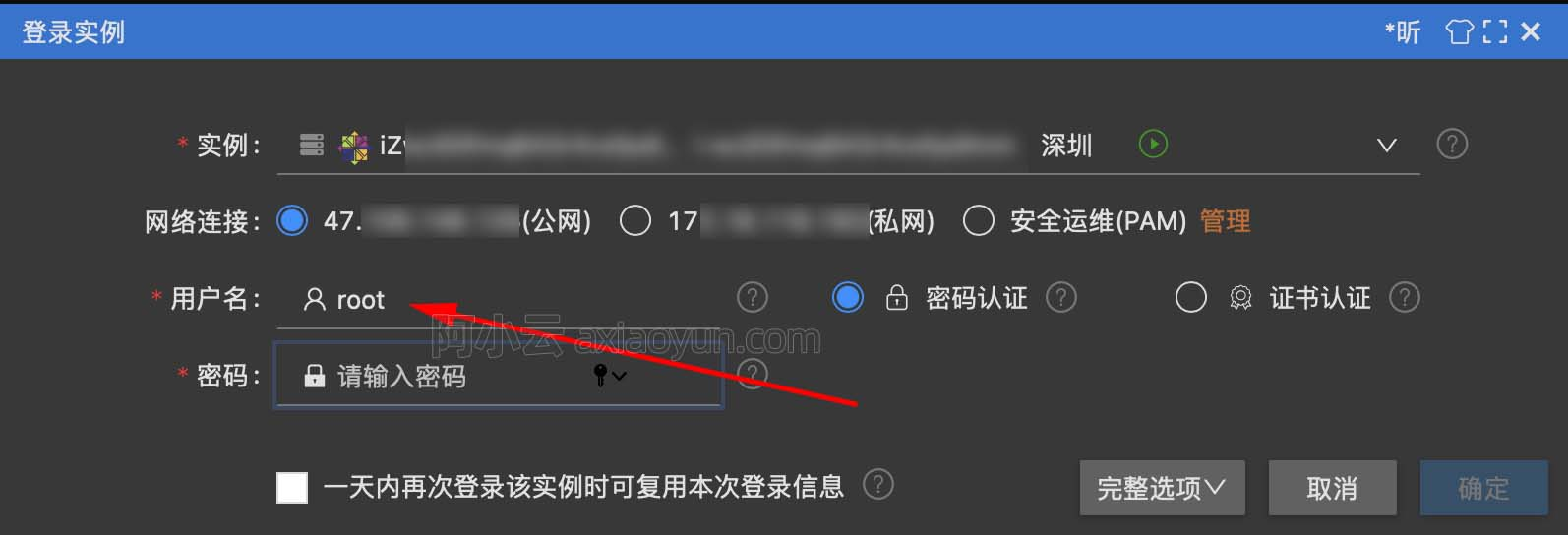+关注继续查看

get(int)，找指定位置上的元素
public E get(int index) {
checkElementIndex(index);
return node(index).item;
}

indexOf(Object)，找元素所在的位置
public int indexOf(Object o) {
int index = 0;
if (o == null) {
for (LinkedList.Node<E> x = first; x != null; x = x.next) {
if (x.item == null)
return index;
index++;
}
} else {
for (LinkedList.Node<E> x = first; x != null; x = x.next) {
if (o.equals(x.item))
return index;
index++;
}
}
return -1;
}

// assert isElementIndex(index);
if (index < (size >> 1)) {
for (int i = 0; i < index; i++)
x = x.next;
return x;
} else {
for (int i = size - 1; i > index; i--)
x = x.prev;
return x;
}
}

for (Iterator<String> it = list.iterator(); it.hasNext();) {

it.next();

}public ListIterator<E> listIterator(int index) {
checkPositionIndex(index);
}
private class ListItr implements ListIterator<E> {
private int nextIndex;
private int expectedModCount = modCount;
ListItr(int index) {
// assert isPositionIndex(index);
next = (index == size) ? null : node(index);
nextIndex = index;
}
public boolean hasNext() {
return nextIndex < size;
}
public E next() {
checkForComodification();
if (!hasNext())
throw new NoSuchElementException();
lastReturned = next;
next = next.next;
nextIndex++;
return lastReturned.item;
}
}

06、总结

https://github.com/itwanger/toBeBetterJavaer9489 026739 06333 014375 0windows server 2008阿里云ECS服务器安全设置

9050 013172 04619 04009 054288 06888 0

1084

0

《2021云上架构与运维峰会演讲合集》

《零基础CSS入门教程》

《零基础HTML入门教程》